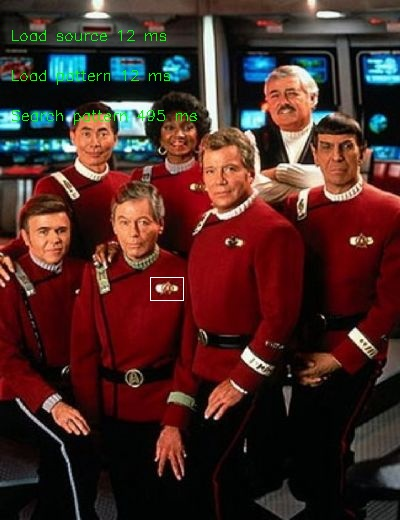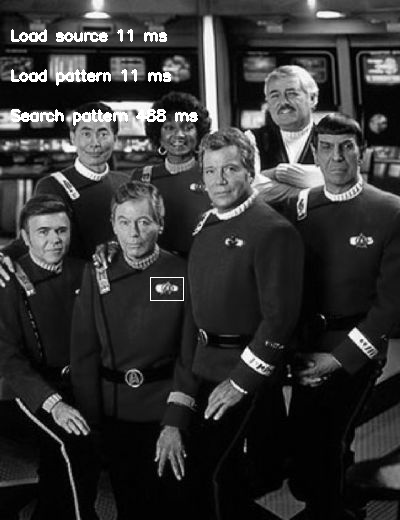## How to search an image inside other image?

Well an interesting thing of computer vision is the possibility of search an image inside other, this process could be used in many systems to search objects, or count (but the images should be to similar and the environment must be under a strict control)

First I took the image to search, in this case is the insignia of doc Mac Coy, just for this exerciseturboStandardUsing gray images directly

In this case the load is a little bit longer in the libjpeg-turbo than the standard one, and the process of find are similar

Here is the code (in the opencv/doc or opencv/samples) there are more examples

To use the function matchTemplate ( imgSrc, imgPattern, .....) both images must be in grayscale, so we can load directly in gray and avoid this step, and we earn 10 ms.

This function, fetch the area were the pattern is in the source.

bool fastMatch (const Mat& _source, const Mat& _pattern,Rect* rectROI, double coincidence)
{

Mat source;
Mat pattern;

Size sourceSize;
Size patternSize;
Size imgResultSize;

Point maxLoc, pointRectROI;
double maxVal;

bool found = false;
// we can avoid this step if we load the image directly in gray
cvtColor(_source,source,CV_BGR2GRAY);
cvtColor(_pattern,pattern,CV_BGR2GRAY);

//We need to take the size of the images.
sourceSize = source.size();
patternSize = pattern.size();

imgResultSize.width = sourceSize.width - patternSize.width + 1;
imgResultSize.height = sourceSize.height - patternSize.height + 1;
Mat imgResult(imgResultSize,CV_32FC1);
//Function that found the image
matchTemplate (source, pattern, imgResult,CV_TM_CCOEFF_NORMED);
minMaxLoc(imgResult,NULL,&maxVal,NULL,&maxLoc);
maxVal *=100;
if (maxVal >= coincidence)
{
*rectROI = Rect(maxLoc.x, maxLoc.y, patternSize.width, patternSize.height);
found=true;
}
return found;
}